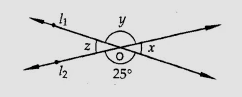"
">

# In the figure, find the values of $x, y$ and $z$."

Given:

Lines $l_1$ and $l_2$ intersect at $O$.

To do:

We have to find the values of $x, y$ and $z$.

Solution:

We know that,

Vertically opposite angles are equal.

Therefore,

$y = 25^o$          (Vertically opposite angles)

$x + y = 180^o$          (Linear pair)

$x + 25^o = 180^o$

$x = 180^o - 25^o$

$x = 155^o$

$z = x = 155^o$          (Vertically opposite angles)

Hence, $x = 155^o, y = 25^o$ and $z = 155^o$.

Updated on: 10-Oct-2022

32 Views